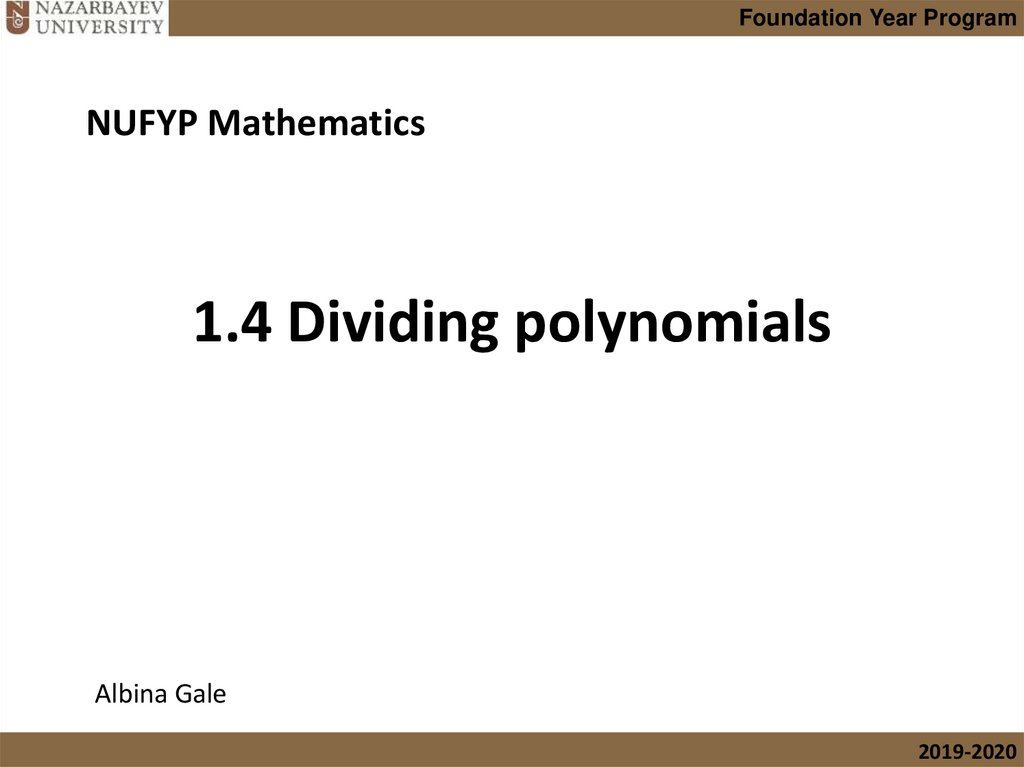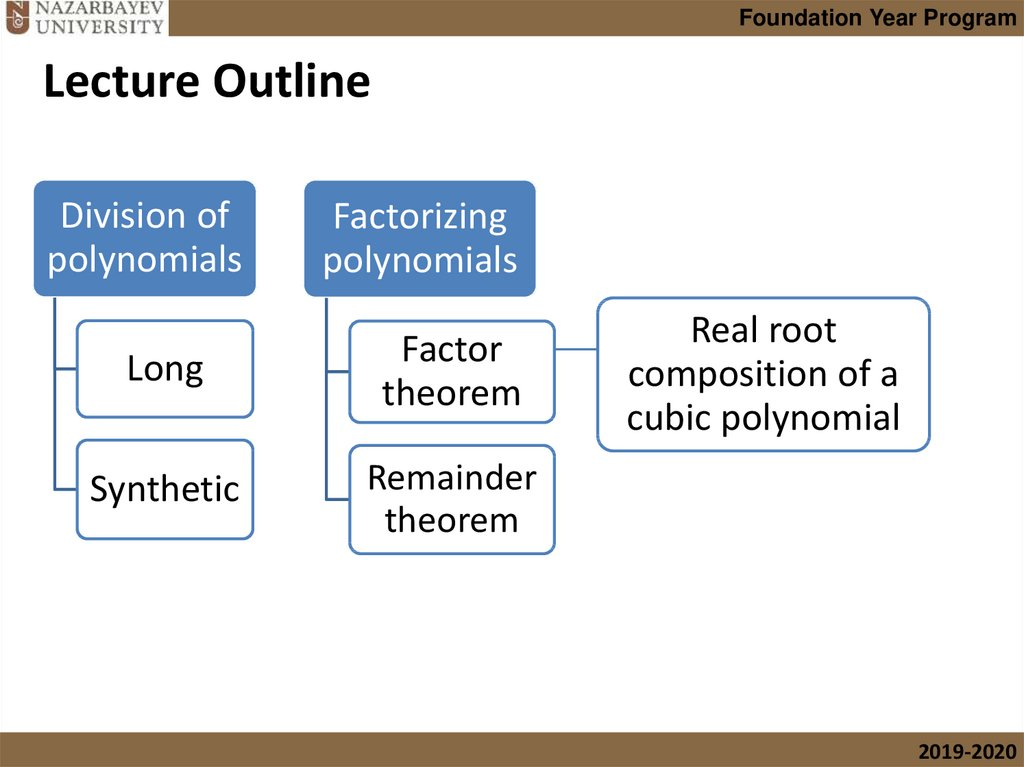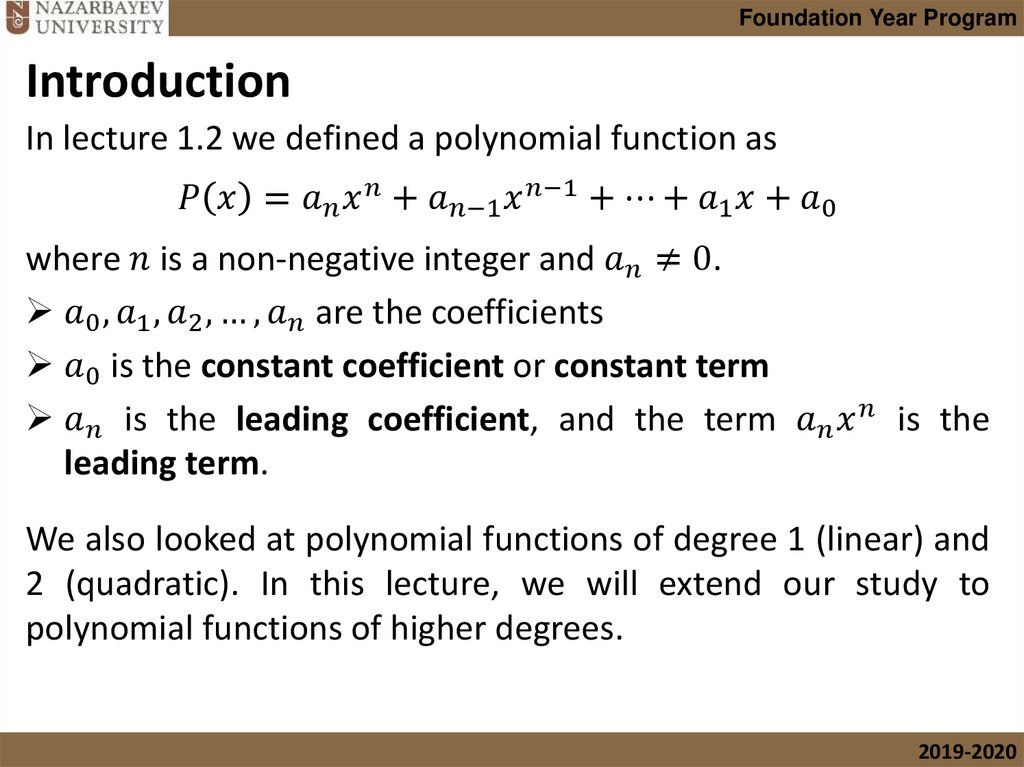# Dividing polynomials

## 1. 1.4 Dividing polynomials

Foundation Year Program
NUFYP Mathematics
1.4 Dividing polynomials
Albina Gale
2019-2020

## 2. Lecture Outline

Foundation Year Program
Lecture Outline
Division of
polynomials
Factorizing
polynomials
Long
Factor
theorem
Synthetic
Remainder
theorem
Real root
composition of a
cubic polynomial
2019-2020

## 3. Introduction

Foundation Year Program
Introduction
In lecture 1.2 we defined a polynomial function as